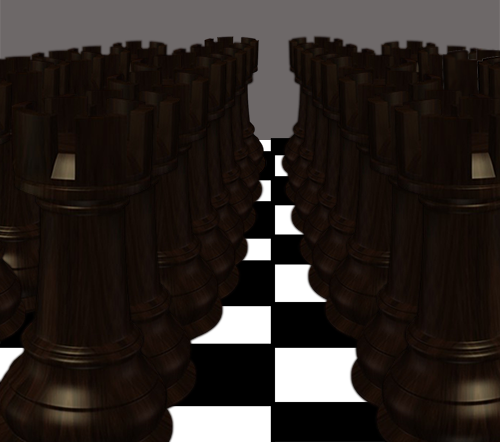# Raging RooksKing Jack wants to prevent enemy pieces from landing on his land. To accomplish this, he will place pieces on as few squares as possible such that every square is attacked, and so any invader will be taken. His piece of choice is the Rook. He already placed a few rooks, but now attacks are becoming more frequent, and he must place more.

His land consists of a $1,000,000\times 1,000,000$ grid of squares, and he currently has $10,000$ rooks already deployed in $10,000$ positions (not necessarily distinct) onto the board. The positions are pairs of integers, $(x_i,y_i)$ with $1\le x_i,y_i\le 1,000,000$. Your task is to find the last three digits of the minimum number of additional rooks Jack needs such that every square on his land is attacked by at least one rook.

Sample input (on a $4\times 4$ grid with 4 rooks already placed)

1 3

2 2

2 4

4 3

Input explanation

The rooks are placed on squares $(1,3)$, $(2,2)$, $(2,4)$, and $(4,3)$.

Answer and explanation

Only $1$ rook is needed, which will be placed at $(3,1)$.

Note that the actual input has 10000 positions.

Details and assumptions

You may find the list of positions here. Each of the 10000 lines contains a space-separated pair of integers $(x_i,y_i)$ that corresponds to the position of a single rook.

A rook attacks every square in the same row as it and/or the same column as it, and so a square with a rook is considered to be attacked.

×

Problem Loading...

Note Loading...

Set Loading...